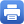Columbia Home
May 01: Gigliola Staffilani (MIT)

Title: The many faces of dispersive equations

Abstract:

In recent years great progress has been made in the study of dispersive and wave equations. The toolbox used in order to attack highly nontrivial problems related to these equations has developed to include a variety of techniques, from Fourier and harmonic analysis to analytic number theory, from math physics to dynamical systems, from probability to symplectic geometry. In this talk I will introduce a variety of problems connected with dispersive and wave equations, such as the derivation of a certain nonlinear Schrodinger equations from a quantum many-particles system, periodic Strichartz estimates, the concept of energy transfer, the non-squeezing theorem for certain dispersive equations viewed as infinite dimension Hamiltonian systems, and if time permits the notion of invariance of a Gibbs measure associated to these systems.

Wednesday, May 01, 4:30 – 5:30 p.m.

Mathematics 520
Tea will be served at 4:00 p.m.Print this page